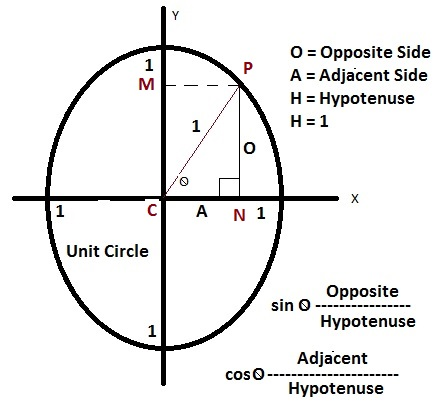# Solving Pythagorean Identities

The Pythagorean identities in trigonometry are the three identities that come from the Pythagorean theorem.

 Enter Angle:

Result

Recall that the Pythagorean theorem states that the hypotenuse squared of a right triangle is the sum of the square of each of the other two sides. a2 + b2 = c2
Where c stands for the hypotenuse, and a and b are other two sides of the right triangle. From this theorem, three identities can be determined from substituting in sine and cosine as follows:
sin2 θ + cos2 θ = 1
tan2 θ + 1 = sec2 θ
1 + cot2 θ = cosec2 θProof of Pythagorean Identities :
Lets drow an unit circle as showing in picture and draw an angle θ since it is a unit circle so line CP = 1, let draw the perpendicual lines to x and y axis as PN and PM.
As we know sinθ = opposite-side(O) / hypotunse and cosθ = adjacent-side (A) / hypotunuse
since hypotunuse = radius = 1 herefore we can write as follows:
sinθ = O and cosθ = A
We know the Pythagorean theorem as CP2 = PN2 + CN2 or 12 = O2 + A2
Now replace the value of O and A with sinθ and cosθ; so we will get
12 = (sinθ)2 + (cosθ)2
1 = sin2θ + cos2θ
sin2θ + cos2θ = 1 --------(1)
To prove other two indentities we can use following formulas:
1 / sinθ = cscθ
1 / cosθ = secθ
sinθ / cosθ = tanθ
cosθ / sinθ = cotθ
let take the basic indentity 1 = sin2θ + cos2θ and device both side by cos2θ
1 / cos2θ = sin2θ / cos2θ + cos2θ / cos2θ
1 / cos2θ = sin2θ / cos2θ + 1
apply basic formulas for 1 / cosθ = secθ and sinθ / cosθ = tanθ, then we will get
sec2θ = tan2θ + 1
1 + tan2θ = sec2θ -------------(2)
again take the basic indentity 1 = sin2θ + cos2θ and device both side by sin2θ
1 / sin2θ = sin2θ / sin2θ + cos2θ / sin2θ
1 / sin2θ = 1 + cos2θ / sin2θ
apply basic formulas for 1 / sinθ = cscθ and cosθ / sinθ = cotθ, then we will get
csc2θ = 1 + cot2θ
1 + cot2θ = csc2θ -------------(3)